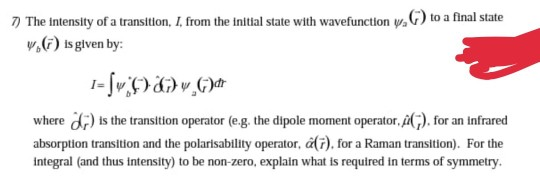# 7) The Intensity of a transition, I, from the initial state with wavefunction w.) to a...The intensity of a transitlon, I, from the initial state with wavefunction $$\psi_{2}(\ddot)$$ to a final state, $$\psi_{b}(\vec{r})$$ is given by:

$$\left.I=\int \psi_{b}^{*}(-) \cdot \hat{\alpha}_{r}\right) \psi_{2}(\vec{r}) d r$$

where $$\vec{d}_{r}^{-}$$) is the transition operator (e.g. the dipole moment operator, $$\hat{\mu}(-)$$, for an infrared absorption transition and the polarisability operator, $$\hat{\alpha}(\vec{r})$$, for a Raman transition). For the integral (and thus intensity) to be non-zero, explain what is required in terms of symmetry.

Initial and final state wavefunctions should have opposite parity i.e opposite symmetry. If one is odd, the other must be even to make any transition possible between these two states. Parity of a wavefunction is determined using the angular momentum quantum number 'L' of the wavefunction. If 'L' is odd 1,3,5..., then wavefunctions have odd parity and if 'L' is even 2,4,6..., then wavefunctions have even symmetry.

#### Earn Coin

Coins can be redeemed for fabulous gifts.

Similar Homework Help Questions